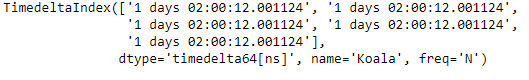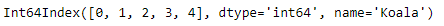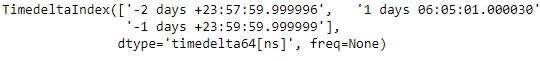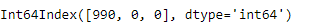# Python | Pandas TimedeltaIndex.nanoseconds

• Last Updated : 29 Dec, 2018

Python is a great language for doing data analysis, primarily because of the fantastic ecosystem of data-centric python packages. Pandas is one of those packages and makes importing and analyzing data much easier.

Pandas` TimedeltaIndex.nanoseconds` attribute return the number of nanoseconds for each element in the TimedeltaIndex object. The value returned ranges from 0 to less than 1 microsecond.

Attention geek! Strengthen your foundations with the Python Programming Foundation Course and learn the basics.

To begin with, your interview preparations Enhance your Data Structures concepts with the Python DS Course. And to begin with your Machine Learning Journey, join the Machine Learning - Basic Level Course

Syntax : TimedeltaIndex.nanoseconds

Return : index object

Example #1: Use `TimedeltaIndex.nanoseconds` attribute to find out the nanoseconds value for each entry of the TimedeltaIndex object.

 `# importing pandas as pd``import` `pandas as pd`` ` `# Create the TimedeltaIndex object``tidx ``=` `pd.TimedeltaIndex(start ``=``'1 days 02:00:12.001124'``, periods ``=` `5``, ``                                            ``freq ``=``'N'``, name ``=``'Koala'``)`` ` `# Print the TimedeltaIndex``print``(tidx)`

Output :Now we will print the number of nanoseconds in each entry of the object.

 `# find nanoseconds number``tidx.nanoseconds`

Output :As we can see in the output, the `TimedeltaIndex.nanoseconds` attribute has returned the number of nanoseconds in each element of the object.

Example #2: Use `TimedeltaIndex.nanoseconds` attribute to find out the nanoseconds value for each entry of the TimedeltaIndex object.

 `# importing pandas as pd``import` `pandas as pd`` ` `# Create the TimedeltaIndex object``tidx ``=` `pd.TimedeltaIndex(data ``=``[``'-1 days 2 min 3us 10ns'``, ``'1 days 06:05:01.000030'``, ``                                                     ``'-1 days + 23:59:59.999999'``])`` ` `# Print the TimedeltaIndex``print``(tidx)`

Output :Now we will print the number of nanoseconds in each entry of the object.

 `# find nanoseconds number``tidx.nanoseconds`

Output :As we can see in the output, the `TimedeltaIndex.nanoseconds` attribute has returned the number of nanoseconds in each element of the object.

My Personal Notes arrow_drop_up